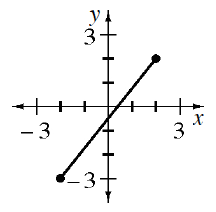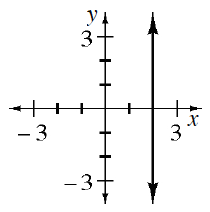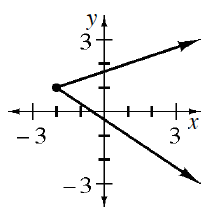### Home > GB8I > Chapter 4 Unit 5 > Lesson INT1: 4.2.3 > Problem4-89

4-89.

For each graph below, what are the domain and range?

See the Math Notes box in Lesson .

1.Domain: $−2\le x\le2$
Range: $−3\le y\le2$

1.Domain: $x=2$
Range: All Numbers

1.1. Which, if any, of the graphs represents a function?

In order for a graph to represent a function, each $x$-value can only relate to one $y$-value.